#Function Repository Resource:

# TanDegree

Compute the tangent of an angle given in degrees

Contributed by: Paco Jain (Wolfram Research)
 ResourceFunction["TanDegree"][x] computes the tangent of an angle x, measured in degrees.

## Details and Options

Mathematical function, suitable for both symbolic and numerical manipulation.
For certain special arguments, TanDegree automatically evaluates to exact values.
TanDegree can be evaluated to arbitrary numerical precision.
TanDegree automatically threads over lists.

## Examples

### Basic Examples

Compute the tangent of an angle:

 In:=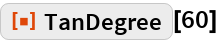Out=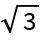Plot over a given range of angles:

 In:=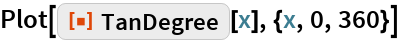Out=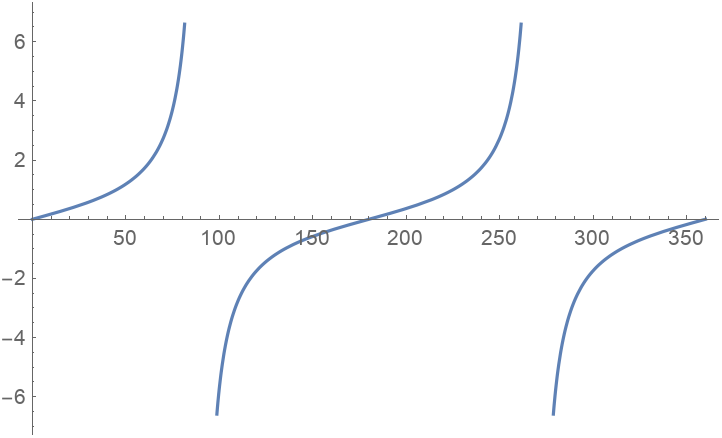### Scope

Evaluate numerically:

 In:=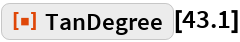Out=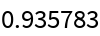Evaluate to high precision:

 In:=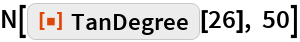Out=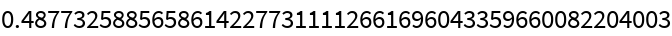## Requirements

Wolfram Language 11.3 (March 2018) or above